### Equilateral Triangle Inscribed In An Equilateral Triangle

Illustration used to prove that triangle EFD is equilateral given that triangle ABC is equilateral and…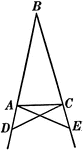### Base Angles In An Isosceles Triangle

Illustration showing that can be used to prove that the base angles of an isosceles triangle are equal.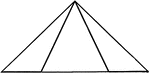### Equal Segments In An Isosceles Triangle

Illustration showing that if equal segments measured from the end of the base are laid off on the base…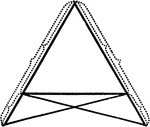### Equal Segments In An Isosceles Triangle

Illustration showing that if equal segments measured from the end of the base prolonged are laid off…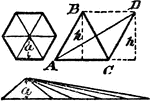### Area Of Regular Polygon Proof

An illustration showing that the area of a regular polygon is equal to the area of a triangle whose…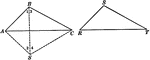### Equal Triangles

Illustration used to show that two triangles are equal if the three sides of one are equal respectively…